This mathematics ClipArt gallery offers 27 Illustrations of planes. A plane is a flat surface with no thickness that is infinitely large. Representations of planes often resemble parallelograms. ClipArt images include intersecting, parallel, and perpendicular planes.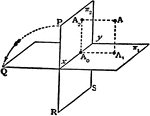### Perpendicular Planes

Two planes lie perpendicular to one another. A line perpendicular to plane 1 and a line perpendicular…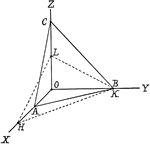### Plane

Illustration showing a how in "analytic geometry it is customary to specify the position of a plane…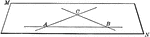### Plane Determined by 2 Intersecting Lines

Illustration of a plane determined by 2 intersecting lines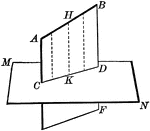### Line Projected Upon Plane

Illustration of a straight line projected upon a plane.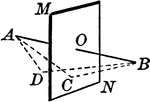### Locus Point to a Plane

"The locus of a point in space equidistant from the extremities of a straight line is the plane perpendicular…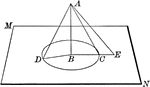### Oblique Lines Drawn to a Plane

Oblique lines drawn from a point to a plane.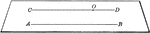### Plane Determined by 2 Parallel Lines

Illustration of a plane determined by 2 parallel lines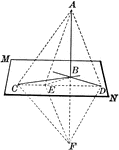### Line Perpendicular to Plane

If a straight line is perpendicular to each of two other straight lines in a plane at their point of…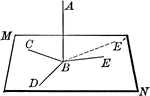### Line Perpendicular to Plane

Straight line AB is perpendicular to the lines in plane MN at point B.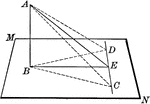### Line Perpendicular to Plane

Straight line AB is perpendicular to the lines in plane MN at point B. "If from the foot of a perpendicular…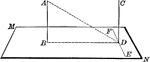### Lines Perpendicular to Plane

Two straight lines, AB and CD, perpendicular to the same plane (MN) are parallel.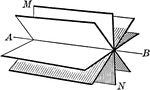### Plane Rotated About a Straight Line

Illustration of a plane rotated about a straight line.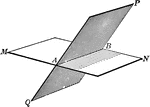### Intersecting Planes

The intersection of two planes is a straight line.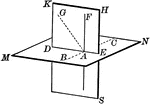### Intersecting Planes

The intersection of two planes is a straight line.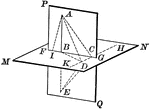### Intersecting Planes

The intersection of two planes is a straight line.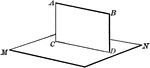### Intersecting Planes

The intersection of two planes is a straight line.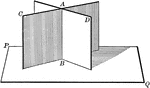### Intersecting Planes

Two intersecting planes on a third plane. "If two intersecting planes are each perpendicular to a third…### Intersecting Planes

Illustration of two intersecting planes.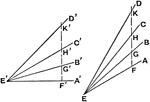### Measuring Angles in Isometric Planes

The illustration showing angles of 15, 30, and 45 degrees at angles 45 and 30 degrees. Measurement of…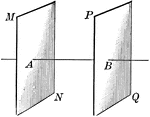### Parallel Planes

Illustration of two parallel planes. "Two planes perpendicular to the same straight line are parallel."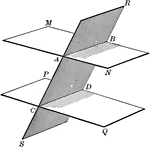### Parallel Planes

Two parallel planes cut by a third plane.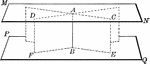### Parallel Planes

Two parallel planes perpendicular to a line (AB). "A straight line perpendicular to one of two parallel…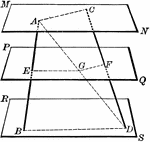### Parallel Planes Cut By 2 Lines

Three parallel planes cut by two lines.### Isometric View of Oblique Timber Framed in to Horizontal Timber

A isometric drawing of the oblique and horizontal wood combined together. The image is drawn by creating…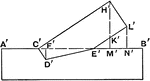### Side View of Oblique Timber Framed in to Horizontal Timber

The illustration of the side view of the oblique timber inserted into the horizontal timber. The drawing…